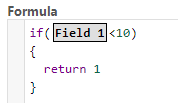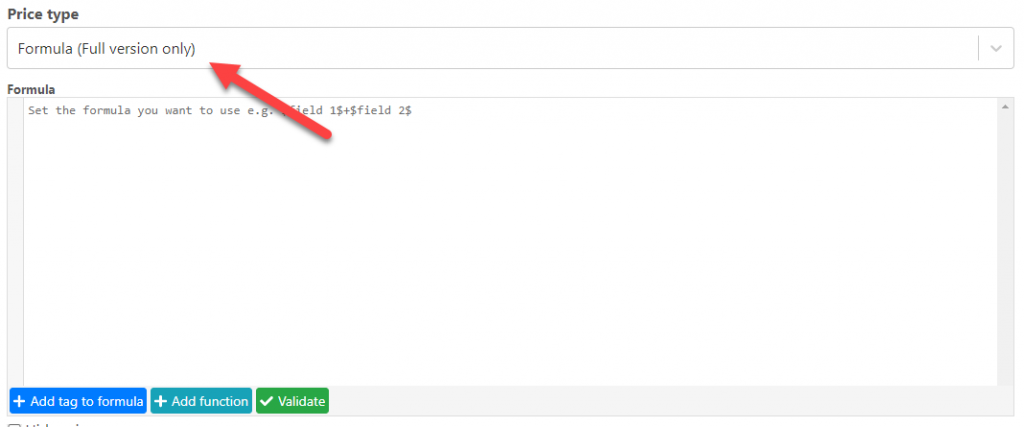## Using Math Functions

Posted by:

Inside a formula, you can type Math. (including the dot) to bring up a list of mathematical functions that you can use

The list includes the number of parameters that the function requires and its explanation, for example, to get the absolute value of a number you can use:

Math.abs(10.11);

## Creating multi-price options

Posted by:

There are different ways to create multi-price options, in this tutorial we are going to cover them

## Changing price using conditional logic

If you just want to use different fixed prices depending on the options selected (you don’t need to do any arithmetical operation to calculate an option price) the best way to do this is by using conditional logic. To change the price with conditions do the following.

1.- Select a field and go to the condition tab

## Using formulas to calculate price for each option

Posted by:

There are many fields (like checkboxes and dropdowns) where you can set a fixed value for each item

But you can also use formulas to change the price of each item dynamically, to do se follow these steps:

1.- Select the price type “Formula Per Item”

2.- Define the formula

3.- That’s it now each option will use that formula to calculate its price

## Field Functions

Posted by:

Some fields have special actions called functions, these functions are used to retrieve specific information from a field. You can see the functions of each field by adding a dot after a field in a formula like this:

\$Field 1\$.

You can use these function anywhere in the formula, for example you could use it in a condition like this:

Posted by:

## Introduction

You can create conditions using an structure like this

``````if(comparison)
{
return value
}
``````

or if you prefer you can use a shorter structure like this:

``````if(comparison)
value``````

Replace “comparison” with the comparison you want and “value” with the value that need to be used, example:In this condition if field 1 has a value less than 10 the value ‘1’ will be used by this formula

You can use multiple if ...

## Introduction

Posted by:

Similar to excel a formula is a way for you to create your own calculations to define things like the price of a product or the text that a box is going to have.You can select the price type “Formula” to create a formula to define the price of a field